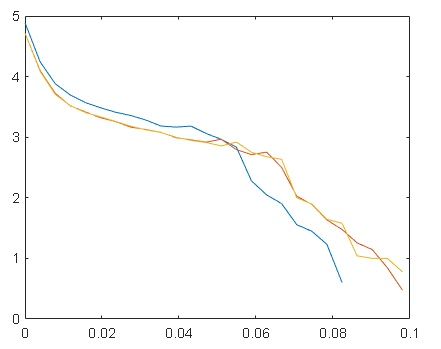# How to evaluate the quality of interpolation? [closed]

I am building a pyramid of images. First I take a big picture and build a smaller one even smaller, etc. I use interpolation to reduce the image. And I need to understand at what interpolation there will be less lost information between images. This is what I mean by interpolation quality. I am looking at horizontal gradients. Please tell me how good this criterion is or if there is something better.

``````Blurred = imfilter(img, PSF);
Blurred = im2double(Blurred)
Blurred2 = imresize(Blurred, [300 300], "Method", "bicubic");
[x0,y0] = meshgrid(1:360,1:360);
[x, y] = meshgrid(1:1.2:360, 1:1.2:360);
Blurred3 = interp2(x0, y0, Blurred, x,y, "spline");
gradX = diff(Blurred,1,1);
gradY = diff(Blurred,1,2);
gradX2 = diff(Blurred2,1,1);
gradY2 = diff(Blurred2,1,2);
gradX3 = diff(Blurred3,1,1);
gradY3 = diff(Blurred3,1,2);
[h, cx]=imhist(gradX);
[h2, cx2]=imhist(gradX2);
[h3, cx3]=imhist(gradX3);
h=log10(h);
h2 = log10(h2);
h3 = log10(h3);
figure, plot(cx, h)
hold on
plot(cx2, h2);
plot(cx3, h3);
hold off
``````## 1 Answer

You're using the finite difference approximation to the derivative. The units in `gradX` are intensity units/pixel, with "pixel" the distance between pixels (which is assumed to be 1). When you rescale your image, you increase the pixel size, but in the derivative you're still assuming the distance between pixels is 1. Thus, the values in `gradX2` are larger than those in `gradX`. You'd have to normalize by the image width to correct for this effect.

But still, after normalization, I don't see how this is a measure of quality of the interpolation. The right question would be: how well can I reconstruct `Blurred` from `Blurred2`? I'm assuming here that `Blurred` has been blurred just sufficiently to avoid aliasing when resampling the image.

I would apply a 2nd round of interpolation to `Blurred2` to recover an image of the same size as `Blurred`, then compare the two images using MSE or similar error measure.

• I'm going to build a pyramid of images. First I take a big picture and build a smaller one even smaller, etc. And I need to understand at what interpolation there will be less lost information between images. This is what I mean by interpolation quality – Denis Tolkachyov Sep 9 '20 at 8:47
• @DenisTolkachyov I understand what you’re doing. If you follow the procedure I’ve outlined you’ll get a good measure of data loss between layers. You can compare to the blurred larger layer, in which case perfect interpolation should give a 0 error, or to the larger layer itself, in which case you’re measuring mostly the loss due to blurring. – Cris Luengo Sep 9 '20 at 18:24